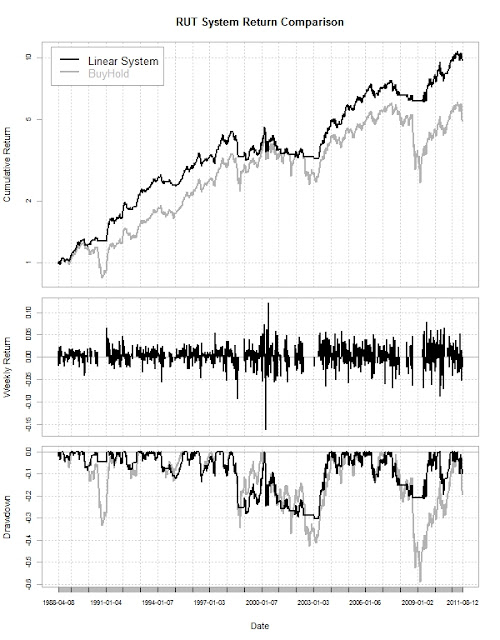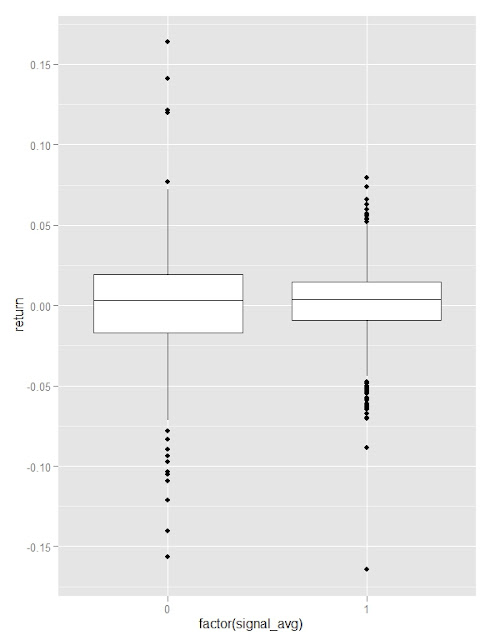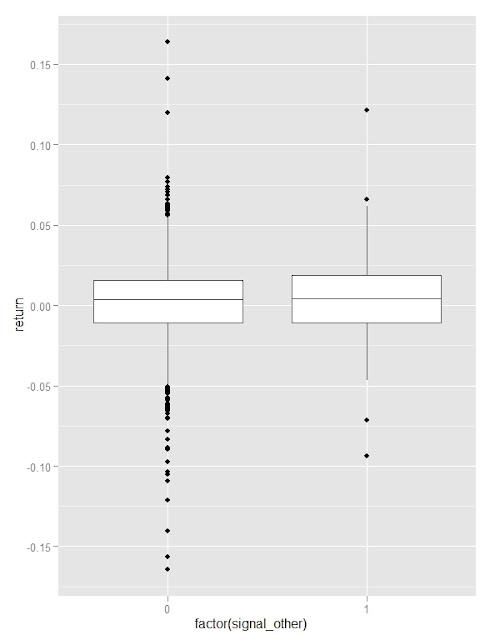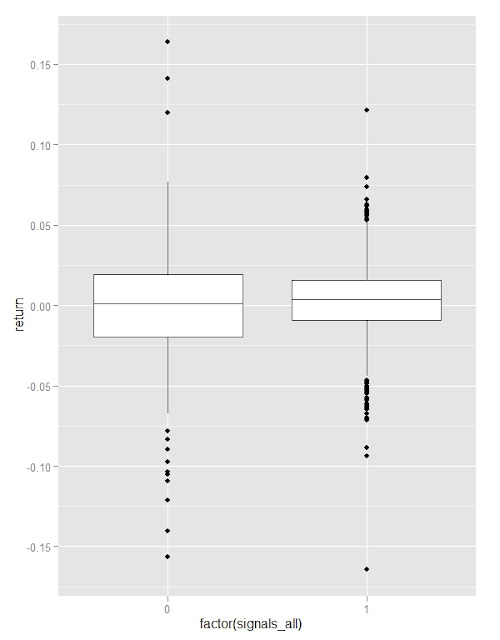Want to share your content on R-bloggers? click here if you have a blog, or here if you don't.

In System Failure-Maybe it Will Help I presented the initial trials of a linear model system for stocks, and even though they were not a resounding success, I have been strangely determined to discover a working version of this framework.  Maybe this blog post John Mayer on Finishing Awful Songs inspired me or maybe I’m just being foolish, but I feel like this framework makes sense.  After way too many hours, I have data mined myself into a much more functional system and one that actually even works on the Russell 2000 which almost always resists submission by my systems as mentioned in Crazy RUT.  Since RUT was the big surprise, I will use it for my charts and analysis, but feel free to use the almost infinite number of indicies from Yahoo! Finance and St. Louis Frederal Reserve FRED.

THIS IS NOT INVESTMENT ADVICE, AND THIS CAN AND PROBABLY WILL LOSE LOTS AND LOTS OF MONEY.From TimelyPortfolioFrom TimelyPortfolioFrom TimelyPortfolioFrom TimelyPortfolioFrom TimelyPortfolio

`#second version#this one actually has an additional mean reverting element#for markets that have moved down so long entry is quicker   require(PerformanceAnalytics)require(quantmod)   #set this up to get either FRED or Yahoo!Finance#getSymbols("RUT",src="FRED")getSymbols("^RUT",from="1896-01-01",to=Sys.Date())     RUT <- to.weekly(RUT)[,4]RUTmean <- runMean(RUT,n=30)#index(RUT) <- as.Date(index(RUT))   width = 10for (i in (width+1):NROW(RUT)) {	linmod <- lm(RUT[((i-width):i),1]~index(RUT[((i-width):i)]))	ifelse(i==width+1,signal <- coredata(linmod\$residuals[length(linmod\$residuals)]),		signal <- rbind(signal,coredata(linmod\$residuals[length(linmod\$residuals)])))	ifelse(i==width+1,signal2 <- coredata(linmod\$coefficients),		signal2 <- rbind(signal2,coredata(linmod\$coefficients)))	ifelse(i==width+1,signal3 <- cor(linmod\$fitted.values,RUT[((i-width):i),1]),		signal3 <- rbind(signal3,cor(linmod\$fitted.values,RUT[((i-width):i),1])))}signal <- as.xts(signal,order.by=index(RUT[(width+1):NROW(RUT)]))signal2 <- as.xts(signal2,order.by=index(RUT[(width+1):NROW(RUT)]))signal3 <- as.xts(signal3,order.by=index(RUT[(width+1):NROW(RUT)]))signal4 <- ifelse(RUT > RUTmean,1,0)   price_ret_signal <- merge(RUT,lag(signal,k=1),	lag(signal2,k=1),lag(signal3,k=1),lag(signal4,k=1),lag(ROC(RUT,type="discrete",n=15),k=1),	ROC(RUT,type="discrete",n=1))price_ret_signal[,2] <- price_ret_signal[,2]/price_ret_signal[,1]price_ret_signal[,3] <- price_ret_signal[,3]/price_ret_signal[,1]   #ret <- ifelse((runMin(price_ret_signal[,3],n=10) >= 0  &#	runSum(price_ret_signal[,2],n=30) >= 0.0) | #	(runMin(price_ret_signal[,3],30) < 0 & #	runSum(price_ret_signal[,2],n=50) >= 0.02),#	 1, 0) * price_ret_signal[,5]#ret <- ifelse(runSum(price_ret_signal[,3],n=10) >= 0, 1, 0) * price_ret_signal[,5]   ret <- ifelse((price_ret_signal[,5] == 1) | (price_ret_signal[,5] == 0  & 	runMean(price_ret_signal[,3],n=50) > 0 & runMean(price_ret_signal[,2],n=10) < 0 ),	1, 0) * price_ret_signal[,7]   retCompare <- merge(ret, price_ret_signal[,7])colnames(retCompare) <- c("Linear System", "BuyHold")#jpeg(filename="performance summary.jpg",#	quality=100,width=6.25, height = 8,  units="in",res=96)charts.PerformanceSummary(retCompare,ylog=TRUE,cex.legend=1.2,	colorset=c("black","gray70"),main="RUT System Return Comparison")#dev.off()   require(ggplot2)df <- as.data.frame(na.omit(merge(price_ret_signal[,5],price_ret_signal[,7])))colnames(df) <- c("signal_avg","return")#jpeg(filename="boxplot by average.jpg",#	quality=100,width=6.25, height = 8,  units="in",res=96)ggplot(df,aes(x=factor(signal_avg),y=return)) + geom_boxplot()#dev.off()   df2 <- as.data.frame(na.omit(merge(ifelse((price_ret_signal[,5] == 0  & 	runMean(price_ret_signal[,3],n=50) > 0 & runSum(price_ret_signal[,2],n=10) < 0 ),	1, 0),price_ret_signal[,7])))colnames(df2) <- c("signal_other","return")#jpeg(filename="boxplot by other signal.jpg",#	quality=100,width=6.25, height = 8,  units="in",res=96)ggplot(df2,aes(x=factor(signal_other),y=return)) + geom_boxplot()#dev.off()   df3 <- as.data.frame(na.omit(merge(ifelse((price_ret_signal[,5] == 1) |	(price_ret_signal[,5] == 0  & 	runMean(price_ret_signal[,3],n=50) > 0 & runMean(price_ret_signal[,2],n=10) < 0 ),	1, 0),price_ret_signal[,7])))colnames(df3) <- c("signals_all","return")#jpeg(filename="boxplot by long signal.jpg",#	quality=100,width=6.25, height = 8,  units="in",res=96)ggplot(df3,aes(x=factor(signals_all),y=return)) + geom_boxplot()#dev.off()   #jpeg(filename="text plot of return and risk.jpg",#	quality=100,width=6.25, height = 6.25,  units="in",res=96)textplot(rbind(table.AnnualizedReturns(retCompare),	table.DownsideRisk(retCompare)[c(1:3,7,11),]))#dev.off()`